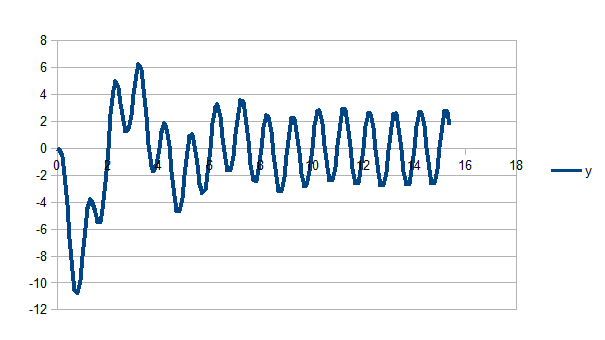# Measuring the natural frequency of a spring-mass system driving force

I'm trying to find a way to use the equation 2.10 but I need ##\theta## and ##A_0##

##\theta## and A are determined by the inital position and velocity. Thus, if at x = 0 the velocity = 0, then A and ##\theta## = 0 ?

haruspex
Science Advisor
Homework Helper
Gold Member
2020 Award
I'm trying to find a way to use the equation 2.10 but I need ##\theta## and ##A_0##
No, you don't. You are only concerned with how the amplitude changes across one cycle, so you can choose the peaks and troughs, i.e. the cos term is +/-1.
If the amplitude at the first trough is ##Ae^{-\Gamma t/2}## then at the next peak it will be ##Ae^{-\Gamma (t+T/2)/2}##, where T is one period of the transient.
What is the ratio of those two expressions? What is that ratio according to my graph?

Do you mean that for low damping ##A = A_0 e^{(-\gamma/2)t}##

The ratio according to the graph seems to be ##-8/-3##, but what this ratio means?

Is it the attenuation per cycle.

Is the attenuation per cycle gamma?

Last edited:
haruspex
Science Advisor
Homework Helper
Gold Member
2020 Award
The ratio according to the graph seems to be ##-8/-3##, but what this ratio means?
Looks to me like the successive extremes are -8, +4, -2, +1... so for one complete cycle the amplitude is multiplied by 1/4.
As to the meaning algebraically, you did not answer this question:
What is the ratio of those two expressions?
... where the two expressions are for the amplitudes half a cycle apart.

##-2e^{(-\gamma(7/2))} / -8e^{(-\gamma/2)}##

But what does that mean?

How you get ##Ae^{-\gamma(t+T/2)/2}##

I still not sure why only this part ##Ae^{-\gamma/2}##.

##Ae^{-\gamma/2}## = ?

Last edited:
haruspex
Science Advisor
Homework Helper
Gold Member
2020 Award
##-2e^{(-\gamma(7/2))} / -8e^{(-\gamma/2)}##

But what does that mean?

How you get ##Ae^{-\gamma(t+T/2)/2}##

I still not sure why only this part ##Ae^{-\gamma/2}##.

##Ae^{-\gamma/2}## = ?
Having obtained the graph of the transient, we can see it oscillates. That means it is underdamped, and alpha in the transient term ##x(t)=Ce^{-\alpha t}## will be complex. This means the transient can be written in the form of eqn 2.11 at the link:
##x(t)=e^{-\Gamma t/2}(c\cos(\omega t)+d\sin(\omega t))##, where ##\omega^2 = \omega_0^2+\Gamma^2/4##.
For our purposes, it is more convenient to write the amplitude as ##C^2=c^2+d^2##, i.e. ##x(t)=Ce^{-\Gamma t/2}(\sin(\omega t+\phi))##.
The graph starts heading down from the origin, so ##\phi=\pi##.
If we focus on the maxima and minima, the sin() will be taking values +1 and -1, and the magnitude is just ##Ce^{-\Gamma t/2}##.
If the period is ##T=\frac{2\pi}{\omega}## and there is a trough at time t0 then the next peak is at time ##t_0+T/2##.
##x(t_0)=-Ce^{-\Gamma t_0/2}##, ##x(t_0+T/2)=Ce^{-\Gamma (t_0+T/2)/2}=Ce^{-\Gamma t_0/2}e^{-\Gamma T/4}##.
So what is ##|\frac{x(t_0+T/2)}{x(t_0)}|##?

Last edited:
•EpselonZero
haruspex
Science Advisor
Homework Helper
Gold Member
2020 Award
It occurred to me that having obtained approximate values for the various frequencies and amplitudes from my crude recreation of the transient, we could model it in a spreadsheet and tune them to get a really good reconstruction of the graph you started with.
So for the transient I plugged in:
- a period of 4 seconds
- a per cycle attenuation of 1/4 (i.e., each complete cycle reduces the amplitude by three quarters),
- an amplitude at one quarter cycle (t=1 second) of -8. (Making C negative meant I could drop the phase shift φ=π.)
and for the steady state portion:
- a period of 1 second
- an amplitude of 2.8 (roughly)
- a phase of 0 (i.e. just sin(wdt)).

With no tweaking at all, it produced:Look familiar?

•EpselonZero
So what is ##|\frac{x(t_0+T/2)}{x(t_0)}|##?

Even with all your explanation I don't know what is that.

##x(t_0) = -Ce^{\Gamma t_0/2}##

If C is the amplitude (-8). Where's x at t=0. In my head at t=0 x = 0.

With the values above did you find ##\omega_0## ?

haruspex
Science Advisor
Homework Helper
Gold Member
2020 Award
Even with all your explanation I don't know what is that.
Just plug in the expressions I gave for x(t0) and x(t0+T/2) in the preceding line.
##x(t_0) = -Ce^{\Gamma t_0/2}##

If C is the amplitude (-8). Where's x at t=0. In my head at t=0 x = 0.

With the values above did you find ##\omega_0## ?
I defined t0 as the time of the first minimum. It is not t=0.
Yes, I found ##\omega_0##.

Just plug in the expressions I gave for x(t0) and x(t0+T/2) in the preceding line.

##\frac{C^e{-\Gamma t_0/2}e^{-\Gamma T/4}}{-Ce^{-\Gamma t_0/2}} = 4/-8##

##\Gamma = 0.69##

Like that?

haruspex
Science Advisor
Homework Helper
Gold Member
2020 Award
##\frac{Ce^{-\Gamma t_0/2}e^{-\Gamma T/4}}{-Ce^{-\Gamma t_0/2}} = 4/-8##

##\Gamma = 0.69##

Like that?
Yes.

So basically, ##\Gamma## is how the system decrease "speed"?

haruspex
Science Advisor
Homework Helper
Gold Member
2020 Award
So basically, ##\Gamma## is how the system decrease "speed"?
It's how the transient dies away, allowing the period to be controlled by the driving force. It continues to affect the amplitude.

I love you! Seriously big big thanks

one more thing if you want. ##A(\omega_d) = 2.8## is that correct?

haruspex
Science Advisor
Homework Helper
Gold Member
2020 Award
I love you! Seriously big big thanks

one more thing if you want. ##A(\omega_d) = 2.8## is that correct?
That's how it looks on the graph you posted.
I encourage you to try to repeat the path I took: observe that the general equation is the sum of a decaying oscillation and a steady oscillation; deduce the steady oscillation parameters from the right hand part of the graph; subtract the equation for the steady part from the graph as a whole to reveal the transient oscillation; from the period and attenuation of the transient deduce its parameters; recreate the curve in a spreadsheet using the parameters calculated.